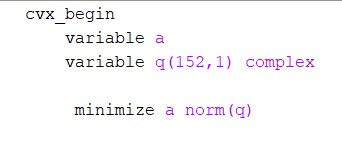# How to minimize two variable?

how to minimize two variable?Not possible with cvx and its solvers. If you decide what exactly you mean by minimizing two objectives, which is not immediately clear, then you can implement it by hand. For example if your intention is to first minimize a, and then among all solutions which achieve that value choose the ones which minimize \|q\| then you can do it exactly in this order by solving two subsequent problems: in the first one you minimize a and in the second you fix a to the value just found and minimize the other objective.

1 Like